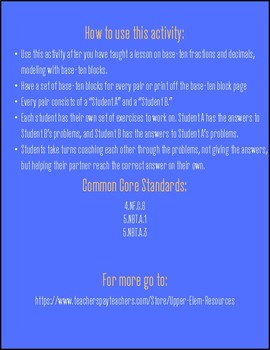# Base-Ten Fractions and Decimals: A Reciprocal Learning ActivitySubject
Resource Type
File Type
PDF (1 MB|11 pages)
Standards
FREE
• Product Description
• Standards
A hands-on reciprocal learning activity where students work with models, turning them into fractions and decimals. This activity will build student's fraction and decimal number sense and conceptual understanding. It will also build fluency in recording numbers different ways.
Use decimal notation for fractions with denominators 10 or 100. For example, rewrite 0.62 as 62/100; describe a length as 0.62 meters; locate 0.62 on a number line diagram.
Use place value understanding to round multi-digit whole numbers to any place.
Recognize that in a multi-digit whole number, a digit in one place represents ten times what it represents in the place to its right. For example, recognize that 700 ÷ 70 = 10 by applying concepts of place value and division.
Total Pages
11 pages